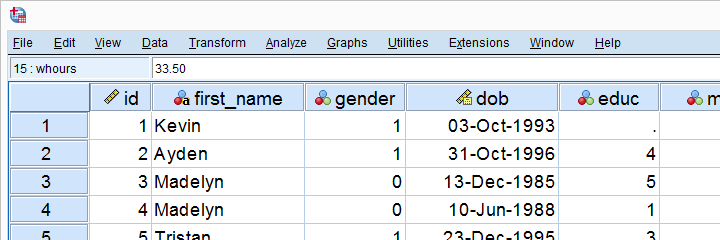SPSS TUTORIALS BASICS ANOVA REGRESSION FACTOR CORRELATION

SPSS Beginners TutorialsIntroduction to SPSS

SPSS – What Is It?

IBM SPSS Statistics (or “SPSS” for short) is super easy software for editing and analyzing data.

This tutorial presents a quick overview of what SPSS looks like and how it basically works.

SPSS Data Editor Window

SPSS’ main window is the data editor. It shows our data so we can visually inspect it.

This tutorial explains how the data editor works: we'll walk you through its main parts and point out some handy tips & tricks.

SPSS Syntax Introduction

SPSS syntax is computer code used by SPSS for analyzing data, editing data, running statistical tests and more.

Using SPSS syntax is super easy and saves tons of time and effort. This tutorial quickly gets you started!

SPSS Output – Basics, Tips & Tricks

SPSS’ output window shows the tables, charts and statistical tests you run while analyzing your data.

This tutorial walks you through some basics such as exporting tables and charts to WORD or Excel. We'll also point out some important tricks such as batch editing and styling tables and charts.

Normality Tests in SPSS

SPSS Shapiro-Wilk Test – Quick Tutorial with Example

The Shapiro-Wilk test examines if a variable is normally distributed in a population. This assumption is required by some statistical tests such as t-tests and ANOVA.

The SW-test is an alternative for the Kolmogorov-Smirnov test. This tutorial shows how to run and interpret it in SPSS.

SPSS Kolmogorov-Smirnov Test for Normality

The Kolmogorov-Smirnov test examines if a variable is normally distributed in some population.

This “normality assumption” is required for t-tests, ANOVA and many other tests. This tutorial shows how to run and interpret a Kolmogorov-Smirnov test in SPSS with some simple examples.

Must-Know Statistics

Effect Size – A Quick Guide

Effect size is an interpretable number that quantifies the difference between data and some hypothesis.

Effect size measures are useful for comparing effects across and within studies. This tutorial helps you to choose, obtain and interpret an effect size for each major statistical procedure.

Cohen’s D – Effect Size for T-Test

Cohen’s D is the effect size measure of choice for t-tests.

This simple tutorial quickly walks you through

• rules of thumb for small, medium and large effects;
• formulas for computing Cohen’s D and;
• software options for obtaining it.

What Does “Statistical Significance” Mean?

Statistical significance is roughly the probability of finding your data under some null hypothesis.

If this probability (or “p”) is low -usually p < 0.05- then your data contradict your null hypothesis. In this case, you conclude that the hypothesis is not true.

Pearson Correlations – Quick Introduction

A Pearson correlation is a number between -1 and +1 that indicates how strongly two variables are linearly related.

This simple tutorial quickly explains the basics with outstanding illustrations and examples.

SPSS - Popular Tutorials

SPSS Missing Values Tutorial

In SPSS, missing values refer to

• system missing values: values that are absent from the data;
• user missing values: values that are present in the data but must be excluded from analyses.

We'll quickly walk you through both types. We'll also show how to detect, set and deal with missing values in SPSS.

SPSS Factor Analysis – Beginners Tutorial

Factor analysis examines which variables in your data measure which underlying factors.

This tutorial illustrates the ideas behind factor analysis with a simple step-by-step example in SPSS.

SPSS Variable Types and Formats

SPSS has 2 types of variables:

• numeric variables contain only numbers and can be used for calculations;
• string variables contain text and cannot be used for calculations.

Numeric variables come in several formats such as plain numbers, dates and percentages. Working with SPSS becomes much faster and easier if you're aware of variable types and formats.

SPSS IF – A Quick Tutorial

In SPSS, IF computes a new or existing variable but for a selection of cases only.

For example: IF(GENDER = 0) SCORE = MEAN(Q1 TO Q5). computes “score” as the mean over variables Q1 to Q5 but only for cases whose gender is 0 (female).

SPSS SELECT IF – Tutorial & Examples

In SPSS, SELECT IF removes a selection of cases from your data.

This tutorial walks you through the basics and some FAQ's such as

• how to remove cases based on 2 variables instead of one?
• how to remove cases based on (number of) missing values?
• how to visually inspect only those cases that will be removed?

SPSS FAQ

How to Find & Exclude Outliers in SPSS?

Three common ways to find outliers are

So how to find precisely which values to exclude? This tutorial walks you through all 3 methods.

Which Statistical Test Should I Use?

The vast majority of statistical tests fall into one of 6 basic types:

Look up which type of test is right for your data and you'll see which test you should use.

How to Convert String Variables into Numeric Ones?

The correct way to convert a string variable into a numeric one is the ALTER TYPE command.

This tutorial walks you through with some examples. We'll point out some tricks, pitfalls and alternatives as well.

Tell us what you think!

*Required field. Your comment will show up after approval from a moderator.

•By Daniel Essiet on June 10th, 2021

•By Ruben Geert van den Berg on June 10th, 2021

Hi Daniel!

Sorry, we don't have a mailing list.

We probably won't publish an awful lot over the next couple weeks due to lots of project work for our students.

However, keep an eye on our facebook page for new tutorials.

Keep up the good work!

SPSS tutorials

•By ISAAC PATRICK on July 30th, 2021

Spss is a nice application i want learn it ....

•By Girma Dersu on July 31st, 2021

I Need this document

•By Ezra Lazarus on August 6th, 2021

It realy help.